# Consider this initial-rate data at a certain temperature for the reaction described by 2NOBr(g) => 2NO(g)

Consider this initial-rate data at a certain temperature for the reaction described by
2NOBr(g) => 2NO(g) +Br2(g)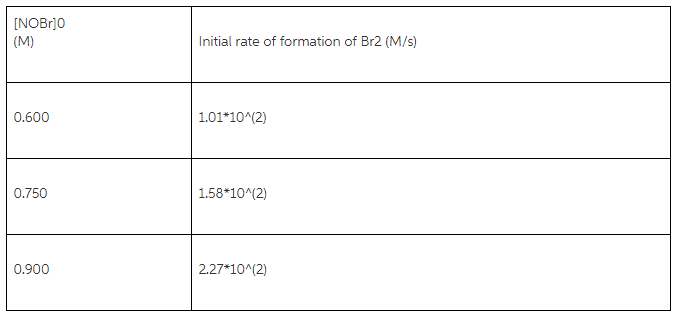Determine the value and units of the rate constant
k =

Concepts and reason
A rate law represents the behavior or nature of a reaction. It describes the relation between the rate of a reaction and the concentrations of reactants.

Fundamentals
Rate law for first order reaction is expressed by: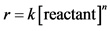Here,k is the rate constant and n is the order of reaction.

For the reaction,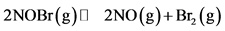The initial rates for formation ofare: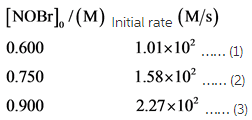Consider the rate law; the rate of gas phase decomposition of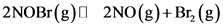This Equation is directly proportional to the concentration of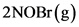.

Substitute the values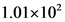for r and 0.600 for reactant in the rate expression in equation 1 as follows: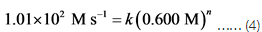Substitute the values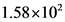for r and 0.750 for reactant in the rate expression for equation 2 as follows: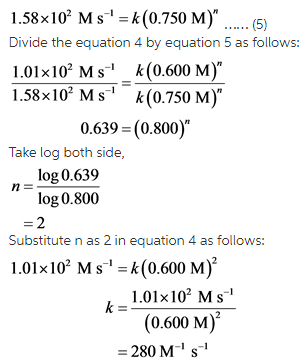The value and unit of rate constant is k is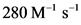Substitute the values of first two equations in the rate expression then, divide the equation to carried out the order of reaction (n). Put the value of n in the first equation of rate expression and the value of k is carried out.